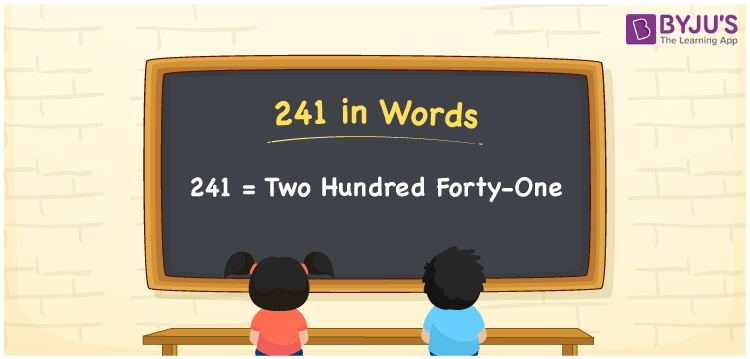# 241 in Words

241 in words can be written as Two Hundred Forty-One. If you pay a toll charge of Rs. 241 at the highways, then you can say that “I paid a toll charge of Two Hundred Forty-One Rupees at the highways”. Using the place value chart curated by the subject matter experts, it will be easy for the students to write the numbers in words with ease. Hence, 241 can be read as “Two Hundred Forty-One” in English.

 241 in words Two Hundred Forty-One Two Hundred Forty-One in Numbers 241

## 241 in English Words## How to Write 241 in Words?

The three column place value chart of 241 is given here to help students learn it easily. With the help of the table, it will be easy for the students to write numbers in words.

 Hundreds Tens Ones 2 4 1

241 in expanded form is shown below:

2 × Hundred + 4 × Ten + 1 × One

= 2 x 100 + 4 x 10 + 1 x 1

= 200 + 40 + 1

= 241

= Two Hundred Forty-One

Therefore, 241 in words is written as Two Hundred Forty-One.

241 is a natural number that precedes 242 and succeeds 240.

241 in words – Two Hundred Forty-One

Is 241 an odd number? – Yes

Is 241 an even number? – No

Is 241 a perfect square number? – No

Is 241 a perfect cube number? – No

Is 241 a prime number? – Yes

Is 241 a composite number? – No

## Frequently Asked Questions on 241 in Words

Q1

### Write 241 in words.

241 can be written as “Two Hundred Forty-One” in words.
Q2

### Write Two Hundred Forty-One in numbers.

Two Hundred Forty-One can be written as 241 in numbers.
Q3

### Is 241 a perfect cube number?

No, 241 is not a perfect cube number as it is not the product of three similar numbers.